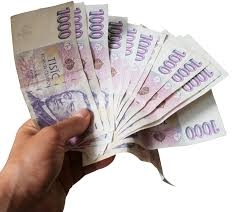# Children 39861

The parents have their 3 children at studies. They cost CZK 3,650 per month. Parents pay 350 CZK less per month for Libor than for Zdenek and 400 CZK more for Eva than for Zdenek. How many CZK per month will he pay for each of them?

L =  850
Z =  1200
e =  1600

### Step-by-step explanation:

L+Z+E=3650
L = Z-350
E = 400 + Z

L+Z+e=3650
L = Z-350
e = 400 + Z

L+Z+e = 3650
L-Z = -350
Z-e = -400

Row 2 - Row 1 → Row 2
L+Z+e = 3650
-2Z-e = -4000
Z-e = -400

Row 3 - 1/-2 · Row 2 → Row 3
L+Z+e = 3650
-2Z-e = -4000
-1.5e = -2400

e = -2400/-1.5 = 1600
Z = -4000+e/-2 = -4000+1600/-2 = 1200
L = 3650-Z-e = 3650-1200-1600 = 850

L = 850
Z = 1200
e = 1600

Our linear equations calculator calculates it.Did you find an error or inaccuracy? Feel free to write us. Thank you!

Tips for related online calculators
Do you have a system of equations and looking for calculator system of linear equations?